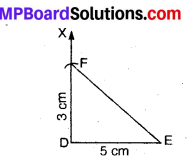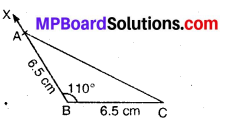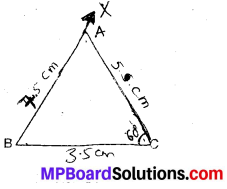## MP Board Class 7th Maths Solutions Chapter 10 Practical Geometry Ex 10.3

Question 1.
Construct ∆DEF such that DE = 5 cm, DF = 3 cm and m ∠EDF = 90°.
Solution:
Steps of Construction :1. Draw a line segment DE = 5 cm.
2. At Q, draw an angle EDX = 90°.
3. From ray DX, cut off DF = 3 cm.
4. Join EF.
Then, DEF is the required triangle.Question 2.
Construct an isosceles triangle in which the lengths of each of its equal sides is 6.5 cm and the angle between them is 110°.
Solution:
Steps of Construction :1. Draw a line segment BC = 6.5 cm.
2. At B draw an ∠CBX =110°.
3. From ray BX, cut off BA = 6.5 cm.
4. Join AC.
Then, ABC is the required isosceles triangle.

Question 3.
Construct ∆ABC with BC = 7.5 cm, AC = 5 cm and m∠C = 60°?
Solution:
Steps of Construction :1. Draw a line segment BC = 7.5 cm.
2. At C, draw ∠BCX = 60°.
3. From ray .CX, cut off CA = 5 cm.
4. Join AB.
Then, ABC is the required triangle.